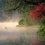# PMI (not neccesarily) Problem

To prove that $\frac{1}{3^2} + \frac{1}{5^2} + \frac{1}{7^2} + \cdots +\frac{1}{(2n+1)^2} < \frac{1}{4}$ for all $n\ belongs\ to\ N$

This was given to be proved by induction in "Self-Learning Exercises - GSEB".Note by Megh Parikh
7 years, 1 month ago

This discussion board is a place to discuss our Daily Challenges and the math and science related to those challenges. Explanations are more than just a solution — they should explain the steps and thinking strategies that you used to obtain the solution. Comments should further the discussion of math and science.

When posting on Brilliant:

• Use the emojis to react to an explanation, whether you're congratulating a job well done , or just really confused .
• Ask specific questions about the challenge or the steps in somebody's explanation. Well-posed questions can add a lot to the discussion, but posting "I don't understand!" doesn't help anyone.
• Try to contribute something new to the discussion, whether it is an extension, generalization or other idea related to the challenge.
• Stay on topic — we're all here to learn more about math and science, not to hear about your favorite get-rich-quick scheme or current world events.

MarkdownAppears as
*italics* or _italics_ italics
**bold** or __bold__ bold
- bulleted- list
• bulleted
• list
1. numbered2. list
1. numbered
2. list
Note: you must add a full line of space before and after lists for them to show up correctly
paragraph 1paragraph 2

paragraph 1

paragraph 2

[example link](https://brilliant.org)example link
> This is a quote
This is a quote
    # I indented these lines
# 4 spaces, and now they show
# up as a code block.

print "hello world"
# I indented these lines
# 4 spaces, and now they show
# up as a code block.

print "hello world"
MathAppears as
Remember to wrap math in $$ ... $$ or $ ... $ to ensure proper formatting.
2 \times 3 $2 \times 3$
2^{34} $2^{34}$
a_{i-1} $a_{i-1}$
\frac{2}{3} $\frac{2}{3}$
\sqrt{2} $\sqrt{2}$
\sum_{i=1}^3 $\sum_{i=1}^3$
\sin \theta $\sin \theta$
\boxed{123} $\boxed{123}$

## Comments

Sort by:

Top Newest

$\frac11^2+\frac13^2+\frac15^2+\dots=\left(\frac11^2+\frac12^2+\frac13^2+\dots\right)-\left(\frac12^2+\frac14^2+\frac16^2+\dots\right)=\frac{\pi^2}{6}-\frac{\pi^2}{4\cdot6}=\frac{\pi^2}{8}$

Hence,

$\frac13^2+\frac15^2+\dots=\frac{\pi^2}{8}-1<\frac14$

Since $f(n)$ is increasing monotonically and the inequality holds asymptotically, the inequality holds for each $n$.

- 7 years ago

Log in to reply

That is exactly my solution above, which is also an overkill (you have to prove $\sum \frac{1}{i^2} = \frac{\pi^2}{6}$, for example).

- 7 years ago

Log in to reply

Overkill: Here $\sum$ indicates $\sum_{i=1}^\infty$. Since $\sum \frac{1}{i^2} = \frac{\pi^2}{6}$, we have $\sum \frac{1}{(2i)^2} = \frac{\pi^2}{24}$. Subtracting the two, we have $\sum \frac{1}{(2i-1)^2} = \frac{\pi^2}{8}$, thus $\sum \frac{1}{(2i+1)^2} = \sum \frac{1}{(2i-1)^2} - \frac{1}{1^2} = \frac{\pi^2}{8} - 1 < \frac{1}{4}$.

I'm pretty sure the method is to figure out some always positive $f(n)$ such that $f(n) - \frac{1}{(2n+1)^2} > f(n+1)$, so we can induct it on $\sum_{i=1}^n \frac{1}{(2i+1)^2} < \frac{1}{4} - f(n)$.

- 7 years, 1 month ago

Log in to reply

Thanks

- 7 years, 1 month ago

Log in to reply

I didn't understand the subtraction of the summation. How did you get the denominator ${(2i-1)}^2$ ? .Could you explain better this part?

- 7 years, 1 month ago

Log in to reply

$\sum \frac{1}{i^2} = \frac{1}{1^2} + \frac{1}{2^2} + \frac{1}{3^2} + \frac{1}{4^2} + \ldots$, and $\sum \frac{1}{(2i)^2} = \frac{1}{2^2} + \frac{1}{4^2} + \frac{1}{6^2} + \ldots$, so subtracting the two gives $\frac{1}{1^2} + \frac{1}{3^2} + \frac{1}{5^2} + \ldots = \sum \frac{1}{(2i-1)^2}$.

- 7 years, 1 month ago

Log in to reply

By guesswork I obtained one f to be $f(n)=\dfrac{n+\frac{19}{16}}{(2n+1)^2}$

- 7 years, 1 month ago

Log in to reply

Explaining my guesswork:

I assumed f to be rational function with denominator to be (2n+1)^2 and numerator to be linear ax+b

Then I got quadratic in n and made it into linear and got a=1.

Then solving for b in term of n, I got b>9/8

Also $f(1) - f(n+1) < 1/4 \implies b<5/4$

So I took b=9.5/8

- 7 years, 1 month ago

Log in to reply

Nicely done.

Note that the second condition is superfluous, because we can choose a different starting point. For example, if we take $b = 2$, then with $g(n) = \frac{n+2}{(2n+1)^2}$, we get that $g(n) - g(n+1) > \frac{ 1}{ (2n+1) } ^2 \text{ for } n \geq 1 .$

It remains to find an $n$ which would give us

$\frac{1}{3^2 } + \frac{1}{5^2} + \ldots + \frac{1}{(2n+1)^2} < \frac{1}{4} - g(n) .$

In this particular case, $n = 5$ works (using a calculator).

Staff - 7 years, 1 month ago

Log in to reply

That is indeed an overkill. The problem is direct if you know that fact, and hence lets look for solutions which do not directly involve it.

Any suggestions for what $f(n)$ could be?

Staff - 7 years, 1 month ago

Log in to reply

×

Problem Loading...

Note Loading...

Set Loading...